# Samacheer Kalvi Books: Tamilnadu State Board Text Books Solutions

## Samacheer Kalvi 10th Maths Chapter 6 Trigonometry Notes PDF Download: Tamil Nadu STD 10th Maths Chapter 6 Trigonometry NotesSamacheer Kalvi 10th Maths Chapter 6 Trigonometry Notes PDF Download: Tamil Nadu STD 10th Maths Chapter 6 Trigonometry Notes

We bring to you specially curated Samacheer Kalvi 10th Maths Chapter 6 Trigonometry Notes PDF which have been prepared by our subject experts after carefully following the trend of the exam in the last few years. The notes will not only serve for revision purposes, but also will have several cuts and easy methods to go about a difficult problem.

 Board Tamilnadu Board Study Material Notes Class Samacheer Kalvi 10th Maths Subject 10th Maths Chapter Chapter 6 Trigonometry Format PDF Provider Samacheer Kalvi Books

## How to Download Samacheer Kalvi 10th Maths Chapter 6 Trigonometry Notes PDFs?

2. Click on the Samacheer Kalvi 10th Maths Notes PDF.
3. Look for your preferred subject.
4. Now download the Samacheer Kalvi 10th Maths Chapter 6 Trigonometry notes PDF.

## Tamilnadu Samacheer Kalvi 10th Maths Solutions Chapter 6 Trigonometry Unit Exercise 6

Question 1.
Prove that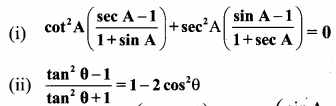Solution: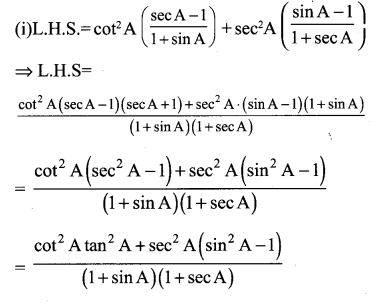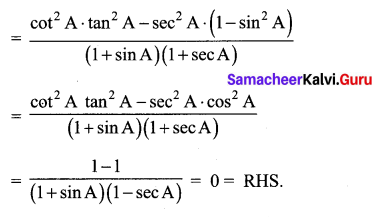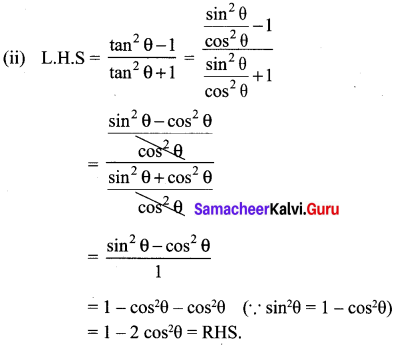Question 2.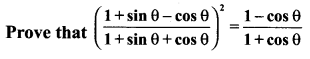Solution: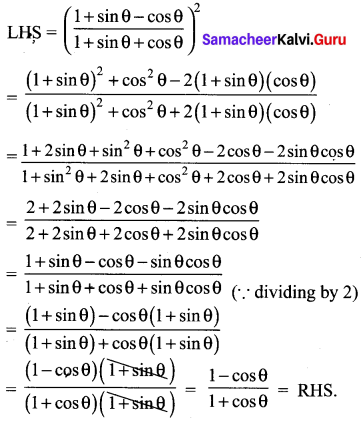Hence proved

Question 3.
If x sin3θ + y cos3θ = sin θ cos θ and x sin θ =
y cos θ , then prove that x2 + y2 = 1.
Solution:
x sin3θ +y cos3θ= sinθ cosθ ; x sinθ y cosθ.
x (sinθ) [sin2θ + cos2θ] = sinθ cosθ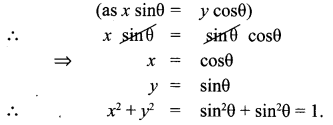Question 4.
If a cos θ – b sin θ = c, then prove that (a sin θ + b cos θ) = ±a2+b2c2−−−−−−−−−−√
Solution: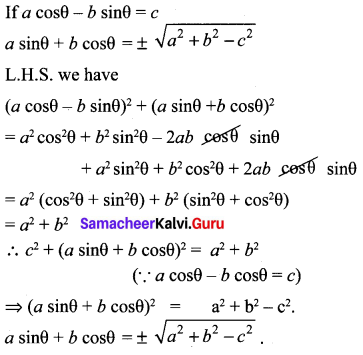Hence Proved.

Question 5.
A bird is sitting on the top of a 80 m high tree. From a point on the ground, the angle of elevation of the bird is 45°. The bird flies away horizontally in such away that it remained at a constant height from the ground. After 2 seconds, the angle of elevation of the bird from the same point is 30°. Determine the speed at which the bird flies. ( 3–√ = 1.732).
Solution:
Let s be the speed of the bird. In 2 seconds, the bird goes from C to D, it covers a distance ‘d’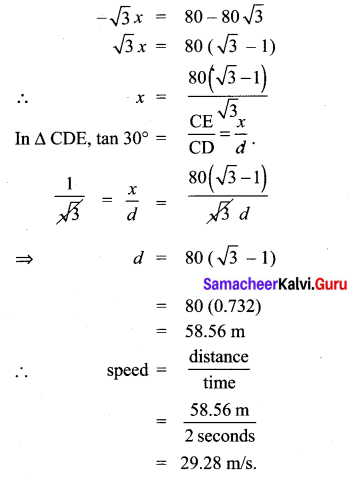Question 6.
An aeroplane is flying parallel to the Earth’s surface at a speed of 175 m/sec and at a height of 600 m. The angle of elevation of the aeroplane from a point on the Earth’s surface is 37° at a given point. After what period of time does the angle of elevation increase to 53°? (tan 53° = 1.3270, tan 37° = 0.7536)
Solution:
Let Plane’s initial position be A. Plane’s final position = D Plane travels from A ➝ D.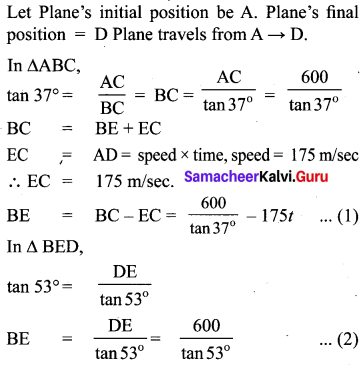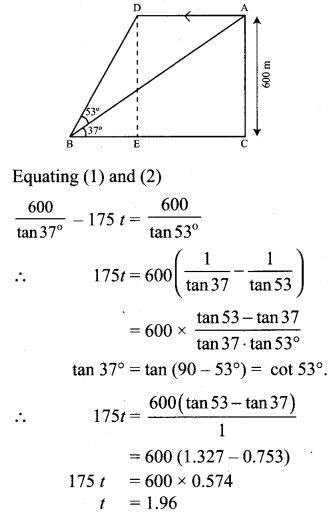Question 7.
A bird is flying from A towards B at an angle of 35°, a point 30 km away from A. At B it changes its course of flight and heads towards C on a bearing of 48° and distance 32 km away.
(i) How far is B to the North of A?
(ii) How far is B to the West of A?
(iii) How far is C to the North of B?
(iv) How far is C to the East of B?
(sin 55° = 0.8192, cos 55° = 0.5736,
sin 42° = 0.6691, cos 42° = 0.7431)
Solution: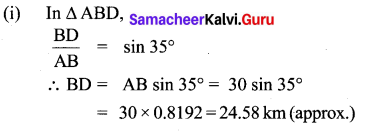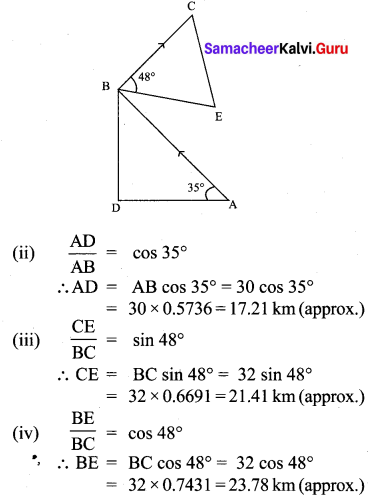Question 8.
Two ships are sailing in the sea on either side of the lighthouse. The angles of depression of two ships as observed from the top of the lighthouse are 60° and 45° respectively. If the
distance between the ships is 200(3+13) metres, find the height of the lighthouse.
Solution:
From the figure AB – height of the light house = h CD – Distance between the ships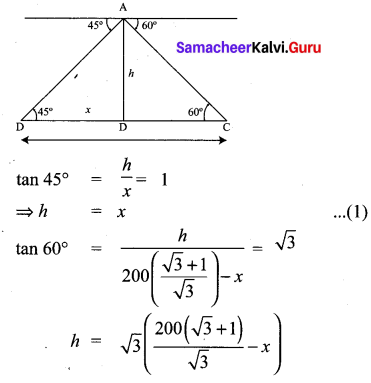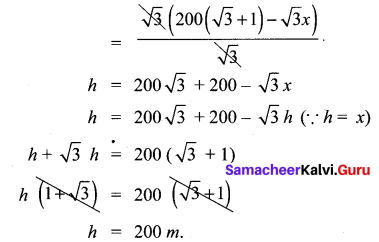∴ The height of the light house is 200 metres.

Question 9.
A building and a statue are in opposite side of a street from each other 35 m apart. From a point on the roof of building the angle of elevation of the top of statue is 24° and the angle of depression of base of the statue is 34°. Find the height of the statue.
(tan 24° = 0.4452, tan 34° = 0.6745)
Solution: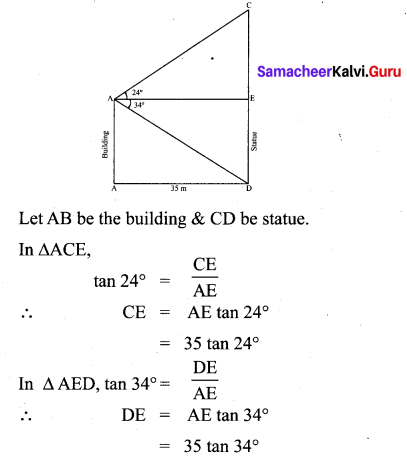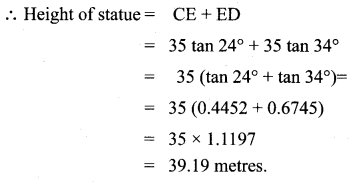## Tamilnadu Samacheer Kalvi 10th Maths Solutions Chapter 6 Trigonometry Additional Questions

Question 1.
Given tan A = 43, find the other trigonometric ratios of the angle A.
Solution:
Let us first draw a right ∆ABC.
Now, we know that tan A = BCAB=43
Therefore, if BC = 4k, then AB = 3k, where k is a positive number.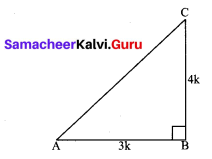Now, by using the pythagoras theorem, we have
AC2 = AB2 + BC2
= (4k)2 + (3k)2 = 25 k2
AC = 5k
So,
Now, we can write all the trigonometric ratios using their definitions.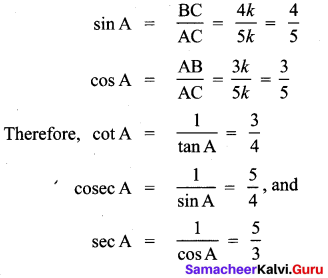Question 2.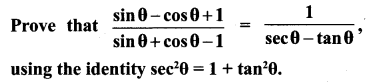Solution:
Since we will apply the identity involving sec θ and tan θ, let us first convert the LHS (of the identity we need to prove) in terms of sec θ and tan θ by dividing numerator and denominator by cos θ.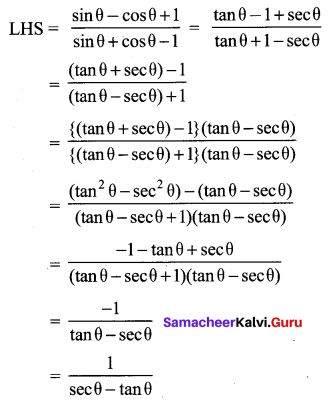Which is the RHS of the identity, we are required to prove.

Question 3.
Prove that sec A (1 – sin A) (sec A + tan A) = 1.
Solution:
LHS = sec A(1 – sin A)(sec A + tan A)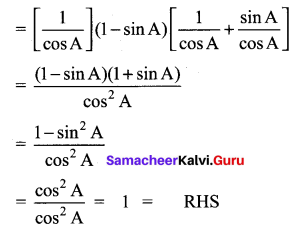Question 4.
In a right triangle ABC, right-angled at B, if tan A = 1, then verify that 2 sin A cos A = 1.
Solution:
In ABC, tan A = BCAB
Let AB = BC = k, where k is a positive number.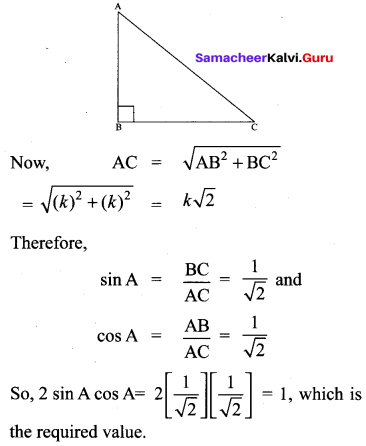Question 5.
If sin (A – B) = 12, cos (A + B) = 12, 0° < A + B ≤ 90°, A > B, find A and BC
Solution: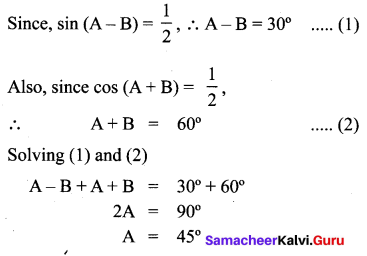We get,
A = 45° and B = 15°

Question 6.
Express the ratios cos A, tan A and sec A in terms of sin A.
Solution:
Since
cos2A + sin2A = 1, therefore,
cos2A = 1 – sin2A
i.e., cos A = ±1sin2A−−−−−−−−√
This gives cos A = 1sin2A−−−−−−−−√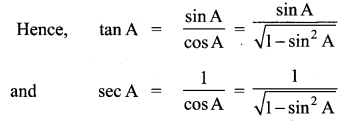Question 7.
Evaluate tan65cot25
Solution:
We know:
cot A = tan(90° – A)
So,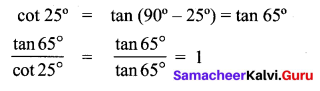Question 8.
Since sin 3A = cos(A – 26°), where 3A is an acute angle, find the value at A.
Solution:
We are given that sin 3A = cos (A – 26°) ….. (1)
Since sin 3A = cos(90° – 3A) we can write (1) as cos(90° – 3A) = cos(A – 26°)
Since 90° – 3A and A – 26° are both acute angles.
90° – 3A = A – 26°
which gives A = 29°

Question 9.
Express cot 85° + cos 75° in terms of trigonometric ratios of angles between 0° and 45°.
Solution:
cot 85° +cos 75°
= cot(90° – 5°) + cos(90° – 15°)
= tan 5° + sin 15°

Question 10.
From a point on a bridge across a river, the angles of depression of the banks on opposite sides at the river are 30° and 45°, respectively. If the bridge is at a height at 3 m from the banks, find the width at the river.
Solution:
A and B represent points on the bank on opposite sides at the river, so that AB is the width of the river. P is a point on the bridge at a height of 3m i.e., DP = 3 m. We are interested to determine the width at the river which is the length at the side AB of the ∆APB.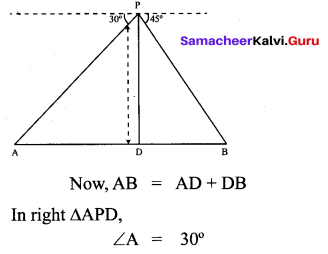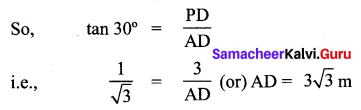Also, in right ∆PBD,
B = 45°
So, BD = PD = 3 m
Now, AB = BD + AD
= 3 + 33–√ = 3(1 + 3–√)m
Therefore, the width at the river is 3(3–√ + 1)

## How to Prepare using Samacheer Kalvi 10th Maths Chapter 6 Trigonometry Notes PDF?

Students must prepare for the upcoming exams from Samacheer Kalvi 10th Maths Chapter 6 Trigonometry Notes PDF by following certain essential steps which are provided below.

• Use Samacheer Kalvi 10th Maths Chapter 6 Trigonometry notes by paying attention to facts and ideas.
• Pay attention to the important topics
• Refer TN Board books as well as the books recommended.
• Correctly follow the notes to reduce the number of questions being answered in the exam incorrectly
• Highlight and explain the concepts in details.

## Frequently Asked Questions on Samacheer Kalvi 10th Maths Chapter 6 Trigonometry Notes

#### How to use Samacheer Kalvi 10th Maths Chapter 6 Trigonometry Notes for preparation??

Read TN Board thoroughly, make separate notes for points you forget, formulae, reactions, diagrams. Highlight important points in the book itself and make use of the space provided in the margin to jot down other important points on the same topic from different sources.

#### How to make notes for Samacheer Kalvi 10th Maths Chapter 6 Trigonometry exam?

Read from hand-made notes prepared after understanding concepts, refrain from replicating from the textbook. Use highlighters for important points. Revise from these notes regularly and formulate your own tricks, shortcuts and mnemonics, mappings etc.
Share: